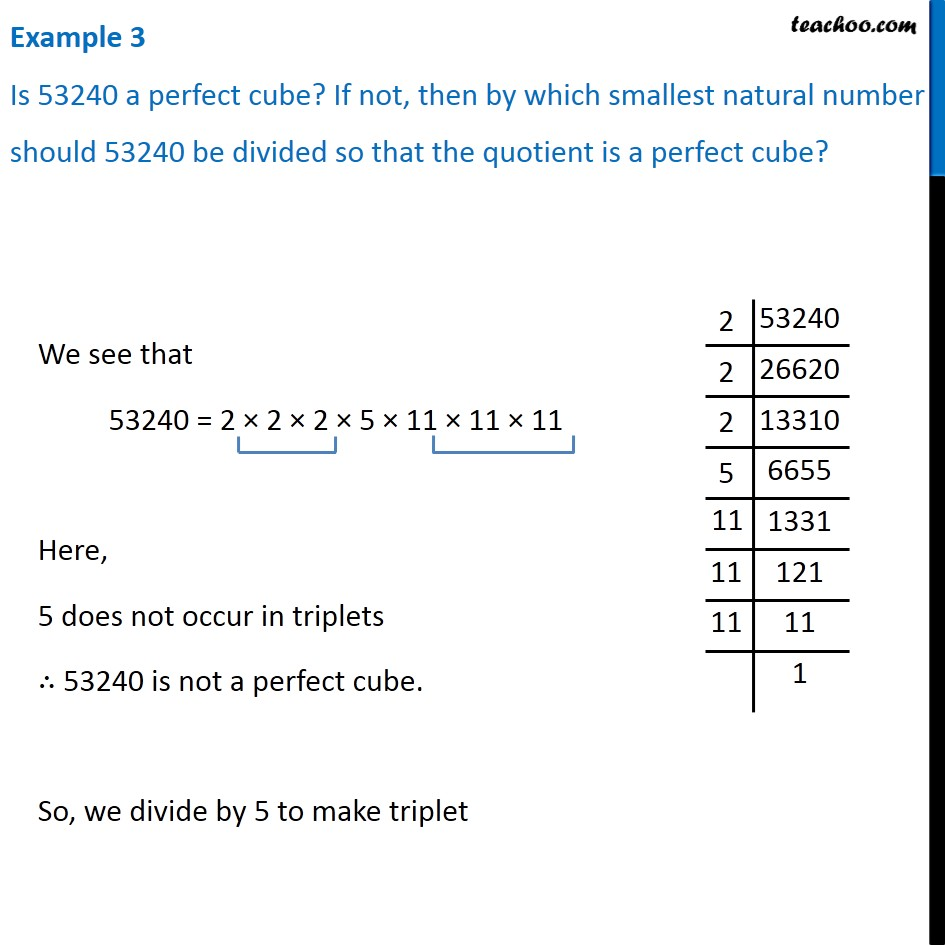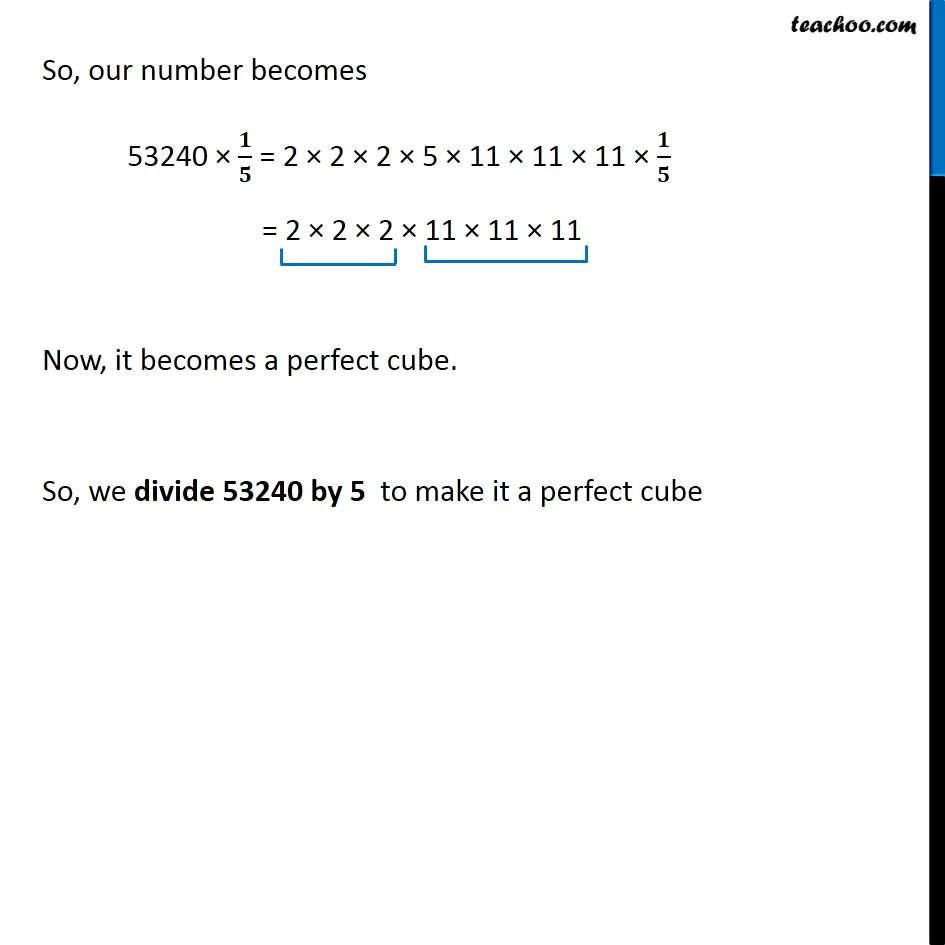Examples

Chapter 6 Class 8 Cubes and Cube Roots
Serial order wiseLearn in your speed, with individual attention - Teachoo Maths 1-on-1 Class

### Transcript

Example 3 Is 53240 a perfect cube? If not, then by which smallest natural number should 53240 be divided so that the quotient is a perfect cube? We see that 53240 = 2 × 2 × 2 × 5 × 11 × 11 × 11 Here, 5 does not occur in triplets ∴ 53240 is not a perfect cube. So, we divide by 5 to make triplet So, our number becomes 53240 × 𝟏/𝟓 = 2 × 2 × 2 × 5 × 11 × 11 × 11 × 𝟏/𝟓 = 2 × 2 × 2 × 11 × 11 × 11 Now, it becomes a perfect cube. So, we divide 53240 by 5 to make it a perfect cube